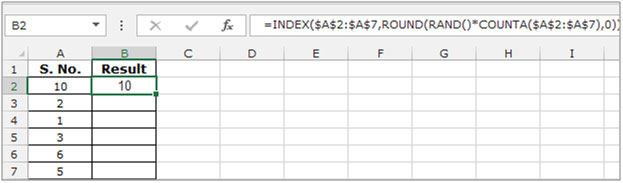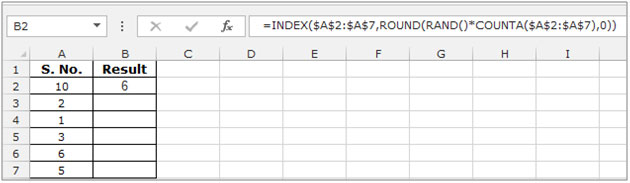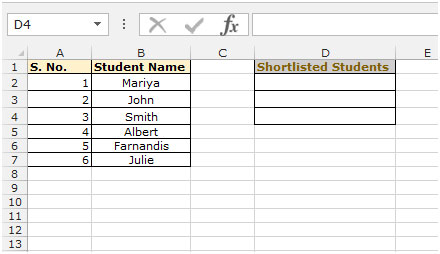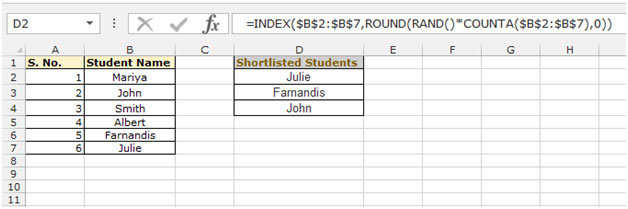# Random name selector

In this article we will learn how to pick the name randomly  from a list, so we will use the INDEX formula along with the ROUND, RAND and COUNTA formulae in Microsoft Excel 2010. We use these functions for Quiz, Games and Lottery etc, where there is no criteria defined for the selection.

Let’s take an example to understand how to select random names from a list in excel:-

Example: We need random number selection i.e. one of the values from the list in column A which contains a list of Serial Nos.

• Select the cell B2, and write the formula
• =INDEX(\$A\$2:\$A\$7,ROUND(RAND()*COUNTA(\$A\$2:\$A\$7),0))
• Press Enter on the keyboard
• The function will return the value 10.• The value will be returned by the formula and will change with each recalculation.
• To understand how it works, select the cell B2 press the key F2 on the keyboard and press enter again.
• The function will return the changed value from the list provided in column A.This is all about that how you can select the random number from a list now we will do a simple exercise to select random text from a list.

We have data in range A1:B7, in which column A contains serial numbers and column B contains the student name. This data belongs to a school, where the application was submitted foradmission. The school is having only 3 seats, while the applicatns are 6. The school’s management wants to select the students randomly without being partial.To randomly select the student name from the list, follow the below given steps:-

• Select the cell D2, and write the formula
• =INDEX(\$A\$2:\$A\$7,ROUND(RAND()*COUNTA(\$A\$2:\$A\$7),0))
• Press Enter on the keyboard.
• The function will randomly pick any name from the list.
• The value will be returned by the formula and will change with each recalculation.
• To randomly select 2 more students from the list copy the formula and paste it into D3 and D4.We would love to hear from you, do let us know how we can improve, complement or innovate our work and make it better for you. Write us at info@exceltip.com

1.The formula works for me. However, I am working from a list of 90 names and I want to choose 45 names. The problem is that there are repeats in 45 names. How do I make sure that once a name is selected, it cannot be selected again?

•Alyson Wright did you figure this out?

•You can do it by having a helper column adjacent to the name column. If you have names in A2:A91 then write =Rand() in B2:B91.
Now in C2 write this formula get unique random names. =INDEX(\$A\$2:\$A\$91,RANK.EQ(B2,\$B\$2:\$B\$91)). Copy down to C46 to get 45 unique random names.

2.Hi Hank,

Use this formula =RANDBETWEEN(A1,25)

3.Hi, I have a row of specific numbers and I want to generate a random number from one of the numbers in that row. Please help. I could do it in Quattro Pro but cannot in Excel.

4.Amazing formula but gives me #VALUE quite often. Specially in short list.

•I experienced the same. I changed to formula to this and haven't seen #VALUE.

=INDEX(\$A\$2:\$A\$7,RANDBETWEEN(1,COUNTA(\$A\$2:\$A\$7)))

Terms and Conditions of use

The applications/code on this site are distributed as is and without warranties or liability. In no event shall the owner of the copyrights, or the authors of the applications/code be liable for any loss of profit, any problems or any damage resulting from the use or evaluation of the applications/code.